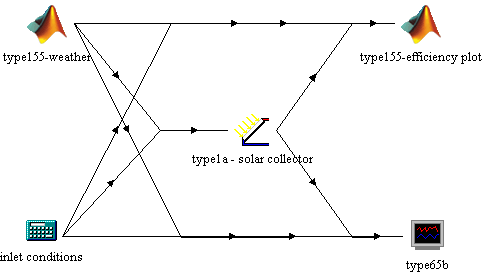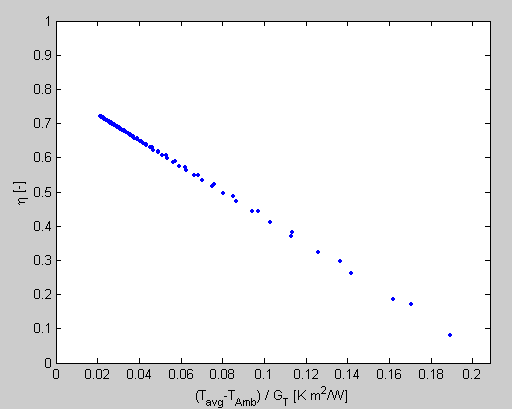Note: Before running this example, you should modify your TRNSYS DLL to include Type155 and the required Matlab libraries, as described in the Type155 manual.

This simple example illustrate the use of 2 Type155 units in a simulation. A simple solar collector (type1b) is simulated with constant inlet conditions and daily sinusoidal ambient temperature and solar radiation. A scatter plot of the collector efficiency is then generated.
• The first unit (type155-weather) generates a sinusoidal profile for ambient temperature and solar radiation based on 3 constant inputs (minimum daily temperature, maximum daily temperature and maximum daily solar radiation)
• The second unit (type155-efficiency plot) will generate a scatter plot of the solar collector efficiency versus (Tavg-Tamb)/GSimulation parameters

• The minimum daily temperature is set by input 1 of "type155-weather"
• The maximum daily temperature is set by input 2 of "type155-weather"
• The maximum daily radiation (in kJ/h/m²) is set by input 3 of "type155-weather"
• The solar collector parameters are set to simulate high efficiency flat-plate collector (1m² area)
• Inlet conditions are set in the equation block

Matlab routines

First unit: Type155-weather

The first Type155 unit has mFileId set to 1, so the following m-files are called by Type155:

• Very first call of the simulation: type155initialize_001.m
• Iterative calls: type155simulate_001.m
• Very last call of the simulation: type155terminate_001.m

ComponentKind is set to 0, which means that Type155simulate_001.m will be called at each iteration of each time step
ignoreEngClose is set to 0, so this routine allows Matlab to be closed when TRNSYS exits

type155initialize_001.m
This routine just stores some information about the unit (nb of inputs, outputs, etc.) and the simulation (nb. of time steps)

type155simulate_001.m
This routine generates sinusoidal profiles for the ambient temperature and the solar radiation using Tamb,min and Tamb,max and Gmax given by type 155 (respectively inputs 1, 2 and 3)

type155terminate_001.m
This routine is empty

Second unit: Type155-efficiency plot

The first Type155 unit has mFileId set to 2, so the following m-files are called by Type155:

• Very first call of the simulation: type155initialize_002.m
• Iterative calls: type155simulate_002.m
• Very last call of the simulation: type155terminate_002.m

ComponentKind is set to 10, which means that Type155simulate_002.m will be called only once per time step. The routine is actually called at the first iteration of a time step with the stored inputs from last call, i.e. the converged inputs from previous time step. This is useful for real-time controllers or for plotting routines, as it is the case here.
ignoreEngClose is set to 1, so this routine does not allow Matlab to be closed when TRNSYS exits. As mentioned in Type155 parameters description, Matlab will keep running after the TRNSYS simulation is done if at least one type 155 unit has this parameter set to 1.

type155initialize_002.m
This routine stores some information about the unit (nb of inputs, outputs, etc.) and the simulation (nb. of time steps). It initializes the history variable, which will be used to store the inputs at each call.

type155simulate_002.m
This routine stores the inputs in the history variable.

type155terminate_002.m
This routine actually draws the plot. It is called at the very last call of the simulation, i.e. after the user presses the "yes" button in the "exit online plotter?" window.
The values corresponding to solar radiation levels lower than 10 W/m² are ignored.

An example plot is given here belowSome TRNSYS-Matlab tips & tricks

Keeping history of inputs/outputs
As always in Matlab, it is a good idea to create history-keeping variables during the initialization call and fill them during the simulation rather than increasing the size of a Matlab variable at each call. This can have a very large effect on the speed of TRNSYS-Matlab simulations.

Troubleshooting m-files
• You can use the Fortran debugger to step through type155 code in order to diagnose prolems in Matlab (when the Fortran debugger stops at some point in Trnsys, you can switch to Matlab and have a look at some variables)
• Unfortunately, the Matlab debugger is not supported in "Matlab Engine Application". You can read article 29983 in the Matlab Solution Base for further information.
• A very useful command is lasterr. This command will tell you the last error that occurred in Matlab. Typically, if an error occurs in an m-file called by Type155, you will hear a series of beeps during the simulation and you will note that some outputs will probably be set to -99999 by type155 (which occurs when the "outputs" variable does not exist or is too short when Matlab returns control to TRNSYS). If you set Type155 not to close the Matlab instance when the simulation is done, you can switch to Matlab, type "lasterr" and see information on the error that occurred
• It is useful to test all m-files with fake input variables from TRNSYS (t, inputs, info) to get rid of the most obvious syntax errors before running them in TRNSYS. This can save you a lot of time and frustration.